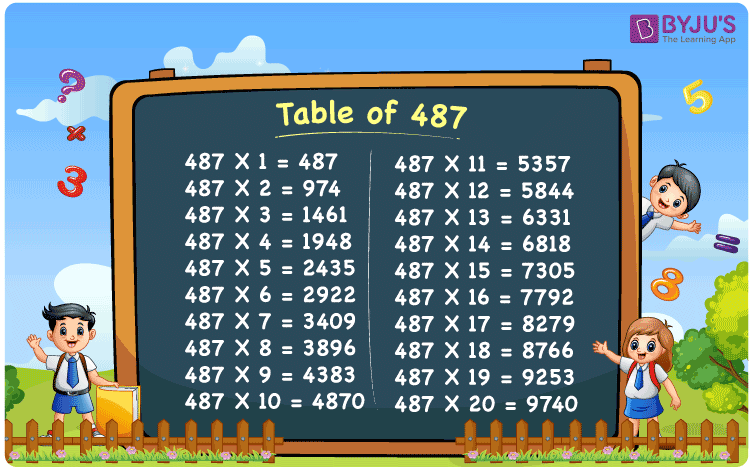Checkout JEE MAINS 2022 Question Paper Analysis : Checkout JEE MAINS 2022 Question Paper Analysis :

# Table of 487

The Table of 487 is the best study material for the students who wish to master the multiplication tables. Multiplication tables form the base of various fundamentals in Mathematics. Those who learn the tables thoroughly will be able to understand the basic concepts effortlessly. With the help of the multiplication table of 487 PDF, scoring good marks in the final exams will be an easy task for the students.

## Table of 487 Chart## What is the 487 Times Table?

The multiplication table of 487 can be used by the students while answering the questions based on the concept of multiplication. Using the chart given below, students will get a clear cut idea about the concept of addition. The main aim of preparing the table is to provide the students with a top notch reference guide, when it comes to exam preparation.

 487 × 1 = 487 487 487 × 2 = 974 487 + 487 = 974 487 × 3 = 1461 487 + 487 + 487 = 1461 487 × 4 = 1948 487 + 487 + 487 + 487 = 1948 487 × 5 = 2435 487 + 487 + 487 + 487 + 487 = 2435 487 × 6 = 2922 487 + 487 + 487 + 487 + 487 + 487 = 2922 487 × 7 = 3409 487 + 487 + 487 + 487 + 487 + 487 + 487 = 3409 487 × 8 = 3896 487 + 487 + 487 + 487 + 487 + 487 + 487 + 487 = 3896 487 × 9 = 4383 487 + 487 + 487 + 487 + 487 + 487 + 487 + 487 + 487 = 4383 487 × 10 = 4870 487 + 487 + 487 + 487 + 487 + 487 + 487 + 487 + 487 + 487 = 4870

## Multiplication Table of 487

The Table of 487 is explained here using various sample questions to provide a better understanding of the concepts. Students who are not familiar with multiplication tables can use these charts and tables for a better understanding. By reading the tables on a daily basis, students will be able to answer the complex questions that are asked in their final exams.

 487 × 1 = 487 487 × 2 = 974 487 × 3 = 1461 487 × 4 = 1948 487 × 5 = 2435 487 × 6 = 2922 487 × 7 = 3409 487 × 8 = 3896 487 × 9 = 4383 487 × 10 = 4870 487 × 11 = 5357 487 × 12 = 5844 487 × 13 = 6331 487 × 14 = 6818 487 × 15 = 7305 487 × 16 = 7792 487 × 17 = 8279 487 × 18 = 8766 487 × 19 = 9253 487 × 20 = 9740

## Solved Example on the Table of 487

Q.1: 20 mangoes were purchased from the shop in a day. How many mangoes will be purchased in 487 days?

Solution: Given,

Number of mangoes purchased in a day = 20

In 487 days number of mangoes that will be purchased = 487 x 20

= 9740

## Frequently Asked Questions on the Table of 487

### Estimate 487 multiplied by 15.

Using the table of 487,
487 multiplied by 15 is 487 x 15 = 7305.

### What is the value of 487 times 5 minus 487 times 9 plus 487 times 3?

In the table of 487,
487 x 5 = 2435
487 x 9 = 4383
487 x 3 = 1461
So we get 487 times 5 minus 487 times 9 plus 487 times 3 = 2435 – 4383 + 1461
= – 487
Hence, the value of 487 times 5 minus 487 times 9 plus 487 times 3 is – 487.

### Find 487 times 17 plus 8 minus 2.

From the table of 487,
487 times 17 plus 8 minus 2 can be written as
487 x (17 + 8 – 2) = 487 x 23
= 11201